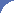# All Science Fair Projects

## Over 500 FREE Science Fair Project Ideas!## The Experiment

Now that you've chosen your question and proposed a hypothesis, the scientific method now requires you to design an experiment to test that hypothesis. If the experiments show that your hypothesis is correct, your hypothesis then becomes scientific theory. If the experiments do not prove your hypothesis, then your hypothesis must be rejected or modified.
How to perform your experiment
An interesting example that you may already know about:-

In the field of astronomy, scientists previously hypothesized that the planets in our galaxy used to circle the earth. Subsequently, through the scientific method, an alternative hypothesis - ie: that the planets actually orbit in concentric circles around the sun - was proved to be correct. Later, this theory was further modified, as the planets' respective orbits were found to be elliptical, and not circular.
As mentioned before, variables are things (or factors) that have an effect on your experiment. There are three kinds of variables that you need to use in your experiment. They are known as independent, dependent, and control variables.
The independent variable
This is the variable that changes throughout the experiment. You will change this variable intentionally in order to see how it affects the other variable known as the dependent variable. In our sample experiment, the SPF rating is the independent variable that would be "changed" by introducing sunscreen lotions of different SPF ratings.
The dependent variable
The dependent variable changes in response to the independent variable. In our example, the level of protection would be the dependent variable, as it would change depending on the SPF rating. At this stage, you will then ask "How do I measure the level of protection?". Your background research will reveal that the level of UV radiation can be measured using a UV meter.
The control variables
The variables that are not changed are called control variables. In the example above, the independent variable is the SPF rating, and the dependent variable is the level of UV radiation measured. Some of the control variables would be the brand of sunscreen lotion used (you should use sunscreens of the same brand, but of different SPF ratings), the quantity of sunscreen lotion used for each experiment, the type of materials and the type of UV meter used for each experiment. In a control, all variables are identical to the original experiment, except for the independent variable. The variables that are identical in both the original experiment and the control are called the control variables.
 Copyright (C) 2014 All Science Fair Projects.com All Rights Reserved. Site All Science Fair Projects Homepage Search | Browse | Links | Handbook | From-our-Editor | Books | Help | Privacy | Disclaimer | Copyright notice